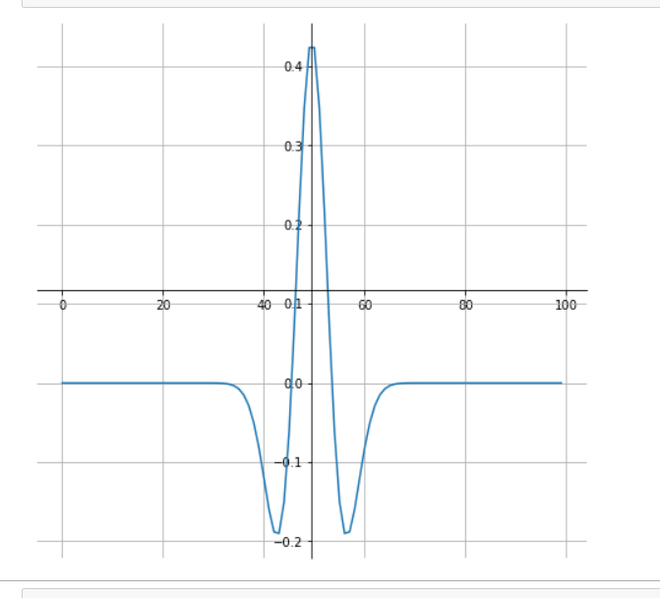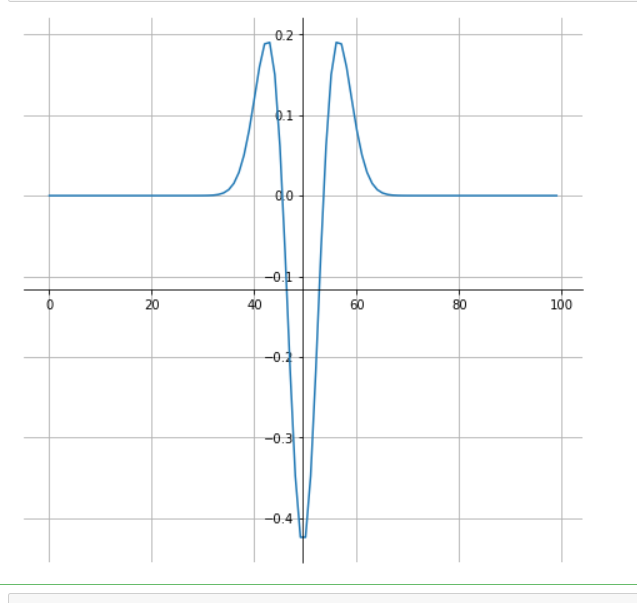# How to plot ricker curve using SciPy – Python?

• Last Updated : 03 Jan, 2021

Prerequisites: Mathplotlib, NumPy

A Ricker wavelet is a wave-like oscillation with an amplitude that begins at 0, increases, and then decreases back to 0. A Ricker wavelet, also known as the “Mexican hat wavelet”.
In this article, we will plot the ricker curve that makes them useful for signal processing. It is the negative normalized second derivative of a Gaussian function.

Syntax: scipy.signal.ricker(points, a)

Parameter:

• points: A number of points in vector. Will be centered around 0.
• a: Width parameter of the wavelet.

Returns: An array of length points in the shape of a ricker curve.

Approach:

• Import required modules.
• Create an array to plot the shape of a ricker curve.
• Move an axis at the center.
• Show Graph.

Example 1: Plotting Ricker curve.

## Python3

 `from` `scipy ``import` `signal``import` `matplotlib.pyplot as plt`` ` `point ``=` `100``hat ``=` `signal.ricker(point, ``4``)`` ` `fig ``=` `plt.figure(figsize ``=` `(``8``, ``8``))``ax ``=` `fig.add_subplot(``1``, ``1``, ``1``)`` ` `# Move left y-axis and bottim x-axis to centre,``# passing through (0,0)``ax.spines[``'left'``].set_position(``'center'``)``ax.spines[``'bottom'``].set_position(``'center'``)`` ` `# Eliminate upper and right axes``ax.spines[``'right'``].set_color(``'none'``)``ax.spines[``'top'``].set_color(``'none'``)`` ` `plt.grid(``True``)``plt.plot(hat)`` ` `plt.show()`

Output:Example 2: Plotting Inverse Ricker curve.

## Python3

 `from` `scipy ``import` `signal``import` `matplotlib.pyplot as plt`` ` ` ` `point ``=` `100``hat ``=` `-``(signal.ricker(point, ``4``))`` ` `fig ``=` `plt.figure(figsize ``=` `(``8``, ``8``))``ax ``=` `fig.add_subplot(``1``, ``1``, ``1``)`` ` `# Move left y-axis and bottim x-axis to centre,``# passing through (0,0)``ax.spines[``'left'``].set_position(``'center'``)``ax.spines[``'bottom'``].set_position(``'center'``)`` ` `# Eliminate upper and right axes``ax.spines[``'right'``].set_color(``'none'``)``ax.spines[``'top'``].set_color(``'none'``)`` ` `plt.grid(``True``)``plt.plot(hat)`` ` `plt.show()`

Output:My Personal Notes arrow_drop_up